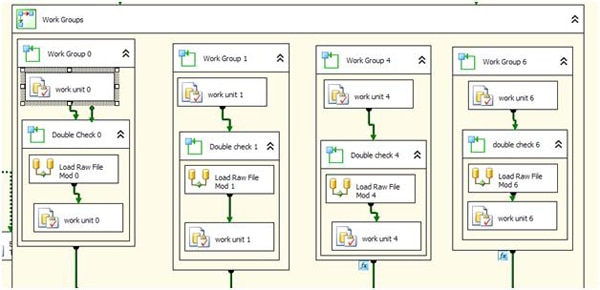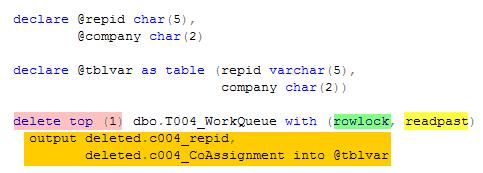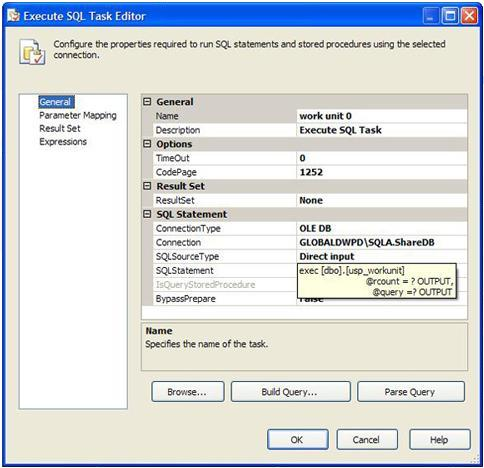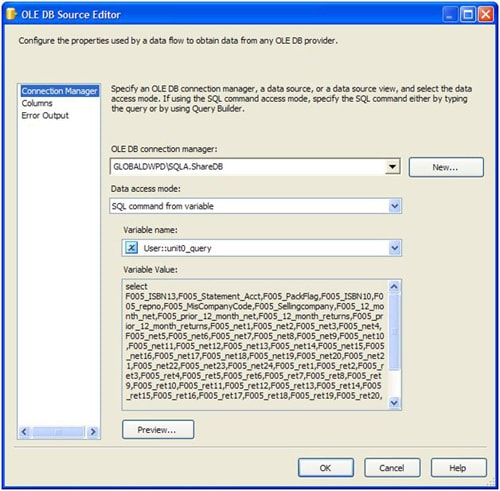# SSIS and Parallelism: The Unseen Minions

Sometimes, a procedural database process cannot easily be reduced to a set-based algorithm in order to reduce the time it takes. Then, you have to find other ways to parallelise it. Other ways? Josef shows how to use SSIS to drastically reduce the time that such a process takes.

In this article, I will show you a real-world program that I built. I took an existing serial program based on stored procedures that ran for 40 hours, and created an SSIS-based one that took 2 hours. To do this, I used parallelism to take advantage of SSIS .

Many developers are aware of the concept of parallelism.  Basically, a parallel system allows me to run multiple units of code simultaneously.   Simplistically, this translates into: “If it took my program 1 hour to run on 1 CPU, it should take 15 minutes to run on 4 CPUs”.  Unfortunately it is not always that simple.  You have to take into account quite a few concepts.  Two important ones  are “unit of work” and “workload distribution”.

# Unit of Work

By ‘Unit of work’, I mean the smallest indivisible amount of work that can be effectively done by an application.   To effectively work in parallel you must be able to divide your work up into smaller pieces and, if possible, more uniform pieces of work.  This is a key part of being able to shift your process from serial to parallel.  If you have large stored procedures which works by dates or, in the case of the original program that I had to deconstruct, salespersons, it took time and patience to identify this ‘unit of work’.  We realized that processing one salesperson at a time was too large a unit and contributed to the reason it took over 40 hours for this process to finish.  I had to dig deeper and see if I could break down the ‘salesperson’ process into something smaller.  You might be thinking ‘Umm, what would be below a salesperson?”  With help from those in the know, it turns out that salespersons are assigned companies that they are allowed to sell to.  It just got more interesting.  My “unit of work” was now ‘SalesPerson/Company’.  Now we were getting somewhere.  I have 2,571 valid combinations of salesperson/company, so for this to work, the total time it takes to run all combinations, must be less than it would to run them  serially or, in the case of SQL Server, in bulk (set theory works on the entire qualifying set).  This doesn’t always work which is why not all processes lend themselves to a parallel workload.  There are many situations where the sum of the parts is greater than the whole.  Let’s see where our threshold for this process is.

The threshold is the point at which a parallel process is no faster than the serial process it is replacing.  First you need to know how long the original process took.  In my case it was 40 hours, which is (40*60) =2,400 minutes.  I have broken out the original process into 2,571 units, so each unit takes on average, 2400/2571= 56 seconds.  Remember since the original process was a bunch of stored procedures which work on the entire data set, this is simply a way to help judge if the units of work and the system that will run your parallel workload are capable of running faster than the original system.

Now I take a random sample of the 2,751 units and run them manually.  Timings show that the average run time is 112 seconds or 2x longer.  How can this be?  There are a number of reasons, first of all this is an average of a random sample you took, not an average of the entire set.  Second, your unit of work might be too large.  There is more work to do in our calculations.

Given that our sample shows 112 seconds serially, this process will take (112*2751)/(60*60)=85 hours.  Hmm, a little more than twice as long to run this process, but we are looking to run this in parallel.  Let’s see how many processes we need to run in parallel to bring the total time down below the original.

• 85/1= 85 hours
• 85/2=42.5 hours
• 85/3=28.3 hours
• 85/4=21.25 hours
• 85/5=17 hours
• 85/6=14.17 hours

As you can see, the more processes you apply, the shorter the total time becomes.  There have been two assumptions in our calculations.  First, we’ve assumed that all units of work take the same average amount of time.  Second, we’ve assumed that the work is distributed evenly across all processes.  There is little that can be done about the first assumption.  Whether you are processing SQL statements (as I am in this example) or you are processing incoming files, it is hard to guarantee that all units of work will take the same amount of time.  Taking the time to figure out a unit of work that is uniform in time is rarely worth the effort.  This can be accomplished by the second assumption: Work is distributed evenly across all processes.

“Workload distribution” is necessary because not every unit of work takes the same amount of time.   Don’t either confuse this with a unit of work or try to define, within your package, a unit of work so that they all take the same amount of time.  This is fruitless.  There are other mechanisms, which I will show you, that compensate for this.  You will undoubtedly have some units of work that take much longer than the average and others that take less time.  This will even itself out without you having to concern yourself with it.  Why be concerned with Workload distribution?  If your longest-running thread takes 1 hour, your package will finish in 1 hour.  This seems reasonable so far, but what if the other 3 threads happen to finish in 15 min? If you do them serially, one after another, you have lost forty-five minutes that I am sure you’d like to reclaim.  To explain how to deal with  unbalanced workloads, I would like to introduce another concept: Silos

# Silos

If you divide up work evenly between threads, by number and not the cost of the unit itself, then you are creating Silos.  I’ve used this technique many times and used it as a springboard to more complex processes, which we will discuss, so let us lay the foundation for that discussion.  Imagine I have 100 units of work that need to be divided up between 4 available threads.  The simplest way to calculate the allocation of  units of work is to  use the modulo arithmetic operator, which finds the remainder of the division of one number by another. 7 mod 4 equals 3.  In SQL Server you would write it like this ‘7%4’. Okaydokay!!.  If we look at the above and rewrite it to be ‘x Mod y’, then we can say “regardless of the value of x your answer will always be between 0 and y-1”.  X mod 4 will return answers in the range of 0-3.  If we take this one step further and make sure the Y is the number of threads you want to build into your SSIS package and we can divide up the work between threads evenly, by number.    You might be asking ‘how do I use this modulo to actually break it up’.  You can use an identity column which simply numbers the records as you push them into the table that you will ultimately retrieve from.   You then do something like this:

The above code will assign each record that equates to a 0 to thread 0.  You would change it to work_number % 4 = 1 and that would assign this record to thread 1 and so on.  One hundred units of work means each thread gets twenty-five units to work on.  If you have an odd number of units (which you might) and an even number of threads (which I prefer to use), then you might have a slightly uneven distribution.  This is where the neat concept of a silo breaks down and you begin to lose the benefits of parallel processing.

Let’s take a less extreme case and say that there are a few poorly performing units of work.  They take twice the average time to run.  If, by a slim chance, these units get funneled into the same thread, that thread’s average run time will begin to creep towards the 2x average and push your entire process to run twice the average unit.  What you really want to do is try to distribute the long running units across all of the threads in order to average them out.  When you use silos, you have two choices:  one is under your control while the other is not.  You can either hope that the law of averages works in your favor or take each unit and run tests and adjust how you calculate how the threads are assigned a unit of work.  I am not in favor of this, because it assumes that the units are static (they ALWAYS take N unit of time and the ones that run long will ALWAYS run long).  Add a unit of work at some point and if that unit takes a very long time, you will have to do some serious re-arranging to prevent it from skewing the entire package.  I prefer semi-intelligent packages.  The other mechanism is the law of averages; not a favorite of mine to rely on.  We’ll use the concept of a queue to help us better distribute work to the threads.

# Queued Work Distribution

Now that you understand the concepts behind parallel work and what we can do in SQL Server and what we should do in SQL Server, let’s work on explaining the package I built and lay the groundwork for you to build your own optimized parallel packages.

# Table based queue structure

As explained above, we are going to build a queue-like structure within SQL Server to help facilitate parallel distribution of work amongst threads.  Let’s start in reverse, by building all of the data structures and then the package structures to take advantage of this work.  You must know what your unit of work is at this point.  I will show you what my unit of work is for the purposes of explaining the concepts.

The unit of work for my package is defined as SalesPerson/Company.  With that in mind, here is the structure of my queue table, yours will be very similar.

This simply lists the combination of SalesPerson to Company, which is our unit of work.  Now we are going to fill this.

I am not going to bore you with the details of how we calculate what SalesPerson can sell to what Company on any given day (yes it can change daily), so you will see a few snippets in this article.

I have a table RepAssignment which holds the SalesPerson/CompanyCode combinations and has a computed column that builds a hash that is based upon the ‘SHA1’formula.   Remember, it’s not enough to have a queue, but you also want to randomize that data going in, to reduce the chance that a single thread will always ask for a ‘long running’ unit.  We do this by using the hashbytes function provided in SQL Server 2005+.  This is function is designed to generate a cryptographic hash based upon the formula and data we provide.  This will provide us with the additional randomness we require to improve the overall performance of our design.  I will go over the concept of cryptographic hash functions in the next section and describe how they help us with randomizing the queue.

We now create a holding temporary table, #hold, which we will use as an interim table to transfer the data from RepAssignment to Workqueue.  What you don’t see in the background is the fact that we are inserting into #hold throughout the procedure as or when different criteria are met.  We do this to consolidate some SalesPerson/Company combinations so as to reduce the number of work units we have to process and in order to improve overall performance.  This will become evident later on in the article as we step through the process by which the SSIS package picks a unit of work and how it is actually executed.

The last step is to insert into the WorkQueue the finalized set of units by HashCode.  This is one more little step to randomize the units.  You will notice that the WorkQueue does not have the HashCode column.  There is no need for it.  We don’t now need to expose the way that we randomized the queue, so long as the data inside the queue has been randomized.  As of now we have a completed queue structure that can be used by an SSIS package.# Hash functions

A hash function is defined by Wikipedia as:

A hash function is any well-defined procedure or mathematical function that converts a large, possibly variable-sized amount of data into a small datum”

A simple hash formula is the Modulo operator.  It takes an arbitrary number of inputs and converts them to a smaller number of outputs.  In the example above, we convert an infinite number of inputs into 4 outputs (0-3).  When you have two or more inputs that convert to the same output, you have what is called a collision.  We are more interested in the distribution of the work.  The higher the collision rate the lower the distribution.  Case in point, with only 4 outputs, a workload of 100 units would distribute something like this 25 units, 25 units,25 units,25 units.  If we add a 101st unit, it would fall in one of those 4 distributions.  Not ideal for randomizing your data.  You want something that provides a more uniform distribution and cryptographic hash functions do that.

A cryptographic hash function is a specific type of hash function defined by Wikipedia as:

A cryptographic hash function is a deterministic procedure that takes an arbitrary block of data and returns a fixed-size bit string, the (cryptographic) hash value, such that an accidental or intentional change to the data will change the hash value”

What this means is that the hash function does such a good job at finding a unique hash that the odds of two totally random input sets will produce the same output set is very, very low.  How low depends upon the function used.   As an example the SHA-1 formula I chose produces a 20 byte(160-bit)  value no matter how small or large (up to 2^64-1 bytes).  A cryptographic hash function is said to have a chance of collision 1 in 2^(<num bits/2) so for SHA-1 it would be 1 in 2^80.  This also means the distribution of the data is more uniform and is not crammed into the 4 distributions using the modulo example.  This more uniform distribution is just what we were looking for to randomize our units of work.

# Dequeue : Getting a unit of work

Now that we have our units or work all lined up and ready to go, we need a thread-safe mechanism to get this work off the queue.  We will use a stored procedure to do this.  A portion of the structure is shown below:

I have highlighted the sections that, when put together, provide a highly scalable and very efficient means of pulling a unit of work off the queue.  We need something that is scalable and efficient.

Traditionally you would issue a begin transaction, set rowcount to 1, update the first record (to get an exclusive lock on it), select the value out of the table, then delete that record, then commit transaction.  Not conducive to parallel work at all.  To make this piece of code and the procedure parallel in nature (by not having this section of code block other spids), we use Delete top(n).

Delete top (1) tells SQL Server to delete the first record in the table.  This not exactly what we want, we want to actually strip that record and use those values.  Using the Output keyword in the delete statement and providing a table variable (@tblvar) we are able to delete a record and capture it in one fell swoop very, very efficient.  We aren’t done yet as there is the matter of concurrency.  There are two table hints available to us that will make this code concurrent in nature rowlock and readpast.  The first hint tells SQL Server to only lock the row it is going to delete, not the entire page.  Since were deleting the top (1) or first row in the table, having a page lock would lock the first ((8192-32) /7) == 1,166 records.  The second hint, readpast, tells SQL Server that if finds a lock on any record it finds, simply move to the next one.  So if there is a request to delete the very first record of the table and another spid comes by it will not wait behind the first request, it will skip past it and delete the 2nd row.  The above code is compact and provides us with robust and parallel capabilities.  We now need to start building the SSIS package framework to enable you to use these structures.

We will begin with what I call the “Engine Housing”.In the package I created there will be 8 simultaneous engines (of which there are 4 showing) running through the units of work.  I say engines, because there is a Data Flow task within each of these and I do not want to confuse you with the parallel concept of threads within there.  Let’s open up one of the engines.  They are all 99% identical, with a few changes to the name and variables that drive them (so they don’t overlap, a concept sometimes lost on those who have never programmed in parallel before).

There are 3 steps:

• Work Unit X: This step pulls the work unit off the queue via stored procedure.
• Double Check X: Makes sure there is work to do
• Load Raw File Mod 0: This step actually ‘runs’ the unit of work.  Don’t be confused with the ‘Mod’ it’s just a hold-over from previous programming.  In this framework we load the result into a raw file for later processing.

# Work Unit X

This task is an Execute SQL task which calls a stored procedure and maps the output to system variables.  The stored procedure call looks like this:There are two output variables, on is used to determine if any work was done, @rcount.  @rcount=1 means I have a unit of work to process.  The unit of work is actually pulled off as a query in the @query string.  The string is then pushed used in a Data Source task where I populate the task using the data access mode: “SQL command from variable”.Let’s take a little deeper look into the work_unit procedure and see exactly what a unit of work is for this package.

# Unit of Work

I designed a unit of work as a select statement, based upon the SalesPerson/Company combination in the WorkQueue table.  The selected columns are the same regardless of the combination.  What changes is the where clause.  In some cases we combine smaller companies into a single select as we have found it is more efficient.  The end result is a fully formed SQL statement that is simply executed by a data source (pictured above).

# Conclusion

We have covered quite a bit of ground in this article.  The intent was to show you how to take hold of the power of threading that SSIS provides natively and use it to your advantage.  I hope this article provided you with enough information to start transforming your own ETL packages into  threaded powerhouses.